Courses

# NCERT Solutions(Part- 3)- Squares and Square Roots Class 8 Notes | EduRev

## Class 8 : NCERT Solutions(Part- 3)- Squares and Square Roots Class 8 Notes | EduRev

The document NCERT Solutions(Part- 3)- Squares and Square Roots Class 8 Notes | EduRev is a part of the Class 8 Course Class 8 Mathematics by VP Classes.
All you need of Class 8 at this link: Class 8

Finding the Square of a number

Example. Find the square of 27.

Solution: (27)= (20 + 7)2

Using the formula (a + b)2 = a+ 2ab + b2, we have

(20 + 7)2 = (20)2 + 2 * (20) * (7) + (7)2

= 400 + 280 + 49

= 729

Thus, (27)2 = 729

Note: For any number ending with 5, the square is a(a + 1) hundred + 25.

For example,

(25)2 = 2(2 + 1) * 100 + 25 = 625

(35)2 = 3(3 + 1) * 100 + 25 = 1225

(65)2 = 6(6 + 1) *100 + 25 = 4225

(125)2 = 12(12 + 1) * 100 + 25 = 15625

Pythagorean Triplets

If three numbers a, b and c are such that a2 + b2 = c2, then they are called Pythagorean Triplets and they represent the sides of a right triangle.

Example:

(i) 3, 4, 5 form a Pythagorean triplet.

[∵ 32 + 42 = 52]

(ii) 8, 15, 17 form a Pythagorean triplet.

[∵ 82 + 152 = 172]

Note: For any natural number n, (n > 1), we have

(2n)2 + (n2 – 1)2 = (n2 + 1)2

such that 2n, n2 – 1 and n2 + 1 are a Pythagorean triplet.

Example. Write a Pythagorean triplet whose one member is 15.

Solution: Since, a Pythagorean triplet is given by 2n, n2 – 1 and n2 + 1.

∴ 2n = 15 or n = 15/2 is not an integer.

So, let us assume that

n2 – 1 = 15

or n2 = 15 + 1 = 16

or n2 = 42, i.e. n = 4

Now, the required Pythagorean triplet is

2n, n2 – 1 and n2 + 1

or   2(4), 4– 1 and 42 + 1

or   8, 15 and 17

Remember

All Pythagorean triplets may not be obtained using the above form.

Question: Find the square of the following numbers containing 5 in unit’s place.

(i) 15 (ii) 95 (iii) 105 (iv) 205

Solution:

(i) (15)2 = 1 * (1 + 1) * 100 + 25

= 1 * 2 * 100 + 25

= 200 + 25 = 225

(ii) (95)2 = 9(9 + 1) * 100 + 25

= 9 * 10 * 100 + 25

= 9000 + 25 = 9025

(iii) (105)2 = 10 * (10 + 1) * 100 + 25

= 10 *11 * 100 + 25

= 11000 + 25 = 11025

(iv) (205)2 = 20 * (20 + 1) * 100 = 25

= 20 * 21 * 100 + 25

= 42000 + 25 = 42025

Exercise 6.2

Que 1. Find the square of the following numbers.

(i) 32 (ii) 35 (iii) 86 (iv) 93 (v) 71 (vi) 46

Solution:

(i) (32)2 = (30 + 2)2

= 302 + 2(30)(2) + (2)2

= 900 + 120 + 4 = 1024

(ii) (35)= (30 + 5)2

= (30)2 + 2(30)(5) + (5)2

= 900 + 300 + 25

= 1200 + 25 = 1225

Second method

352 = 3 * (3 + 1) * 100 + 25

= 3 * 4 * 100 + 25

= 1200 + 25 = 1225

(iii) (86)2 = (80 + 6)2

= (80)2 + 2(80)(6) + (6)2

= 6400 + 960 + 36 = 7396

(iv) (93)2 = (90 + 3)2

= (90)2 + 2(90)(3) + (3)2

= 8100 + 540 + 9 = 8649

(v) (71)2 = (70 + 1)2

= (70)+ 2(70)(1) + (1)2

= 4900 + 140 + 1 = 5041

(vi) (46)2 = (40 + 6)2

= (40)2 + 2(40)(6) + (6)2

= 1600 + 480 + 36 = 2116

Que 2. Write a Pythagorean triplet whose one member is

(i) 6 (ii) 14 (iii) 16 (iv) 18

Solution:

(i) Let 2n = 6       ∴n = 3

Now, n2 – 1 = 32 – 1 = 8

and n2 + 1 = 32 + 1 = 10

Thus, the required Pythagorean triplet is 6, 8, 10.

(ii) Let 2n = 14        ∴ n = 7

Now, n2 – 1 = 72 – 1 = 48

and n2 + 1 = 72 + 1 = 50

Thus, the required Pythagorean triplet is 14, 48, 50.

(iii) Let 2n = 16      ∴n = 8

Now, n2 – 1 = 82 – 1

= 64 – 1 = 63

and n2 + 1 = 82 + 1

= 64 + 1 = 65

Thus, the required Pythagorean triplet is 16, 63, 65.

(iv) Let 2n = 18          ∴n = 9

Now, n2 – 1 = 92 – 1

= 81 – 1 = 80

and n+ 1 = 92 + 1

= 81 + 1 = 82

Thus, the required Pythagorean triple is 18, 80, 82.

 Q. Write all the square numbers between 100 and 300.Ans: 121, 144, 169, 196, 225, 256 and 289  Q. Which of the following would end with digit 9: 1232, 772, 842, 1612, and 102. Ans: (123)2 and (77)2Q. Write all the non-square numbers between 42 and 52.Ans: 17, 18, 19, 20, 21, 22, 23 and 24 Q. Fill in the blanks:(i) 1 + 3 + 5 + 7 + 9 + … = 52(ii) 1 + 3 + 5 + 7 + 9 + … = 62Ans: (i) 11 (ii) 11 + 13 Q. Fill in the blanks: (i) 112 = …… + …… (ii) (…..)2 = 112 + 113 Ans: (i) 60 + 61 (ii) (15)2Q. Find the square of the following numbers actual multiplication: (i) 39 (ii) 42Ans: (i) 1521 (ii) 1764Q. Find the square of: (i) 35 (ii) 105 Ans: (i) 1225 (ii) 11025Q. Write the Pythagorean triplet whose smallest number is 8.Ans: 8, 15, 17Q. Find a Pythagorean triplet in which one member is 12.Ans:  12, 35, 37

Square Roots

Finding the square root of a number is just the opposite operation of squaring it. For example, the square of 5 is 25.

∴ Square root of 25 is 5.

Note: We kwon that (–2) * (–2) = 4, then we say that (–2) is also the square root of 4. Similarly, square roots of 100 are 10 and (–10).

But in this chapter, we shall be studying about positive square roots only

Finding Square Root Through Prime Factorisation

We know that a factor that occurs once in the prime factorisation of a number, occurs twice in the prime factorisation of its square. Thus, we can use this fact of prime factorisation of a number to find the square root of a perfect square.

Note: A perfect square has complete pairs of its prime factors.

Example. Is 1008 a perfect square? If not, find the smallest multiply of 1008 which is a perfect square end then find the square root of the new number.

Solution: We have

1008 = 2 * 2 * 2 * 2 * 3 * 3 * 7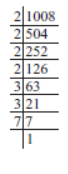As the prime factor 7 has no pair.

∴1008 is not a perfect square. Obviously, if 7 gets a pair, then the number will become a perfect square.

∴1008 * 7 = [2 * 2 * 2 * 2 * 3 * 3 * 7] * 7

or  7056 = 2 * 2 * 2 * 2 * 3 * 3 * 7

Thus, 7056 is the required multiple of 1008 which is a perfect square.

Now,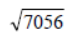= 2 * 2 * 3 * 7 = 84

Question: (i) 112 = 121. What is the square root of 121?
(ii) 142 = 196. What is the square root of 196?

Solution: (i) The square root of 121 is 11.
(ii) The square root of 196 is 14.

Think, Discuss and Write

Question:

(–1)2 = 1. Is –1, a square root of 1?

(–2)2 = 4. Is –2, a square root of 4?

(–9)2 = 81. Is –9, a square root of 81?

Solution:

(i) Since (–1) * (–1) = 1

i.e. (–1)2 = 1

∴ Square root of 1 can also be –1.

Similarly,

(ii) Yes (–2) is a square root of 4.

(iii) Yes (–9) is a square root of 81.

Since, we have to consider the positive square roots only and the symbol for a positive square
root is √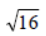= 4 [and not (–4)]

Similarly,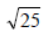means, the positive square root of 25, i.e. 5.

Note: We can also find the square root by subtracting successive odd numbers starting from 1.

Question: By repeated subtraction of odd numbers starting from 1, find whether the following numbers are perfect squares or not? If the number is a perfect square, then find its square root.

(i) 121 (ii) 55 (iii) 36 (iv) 49 (v) 90

Solution:

(i) Subtracting the successive odd numbers from 121, we have

121 – 1 = 120          120 – 3 = 117

117 – 5 = 112          112 – 7 = 105

105 – 9 = 96            96 – 11 = 85

85 – 13 = 72            72 – 15 = 57

57 – 17 = 40            40 – 19 = 21

21 – 21 = 0

∴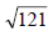= 11. [∵ We had to subtract the first 11 odd numbers.]

(ii)

∵ 55 – 1 = 54    54 – 3 = 51
51 – 5 = 46    46 – 7 = 39
39 – 9 = 30    30 – 11 = 19
19 – 13 = 6    6 – 15 = –9

and we do not reach to 0. ∴ 55 is not a perfect square.

(iii)

∵ 36 – 1 = 35    35 – 3 = 32
32 – 5 = 27    27 – 7 = 20
20 – 9 = 11    11 – 11 = 0

and we have obtained 0 after subtracting 6 successive odd numbers.

∴ 36 is a perfect square.

Thus,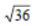= 6.

(iv) We have

49 – 1 = 48       48 – 3 = 45
45 – 5 = 40       40 – 7 = 33
33 – 9 = 24       24 – 11 = 13
13 – 13 = 0

∵ We have obtained 0 after successive subtraction of 7 odd numbers.

∴ 49 is a perfect square,

Thus,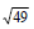= 7.

(v) We have:

90 – 1 = 89       89 – 3 = 86
86 – 5 = 81       81 – 7 = 74
74 – 9 = 65       65 – 11 = 54
54 – 13 = 41     41 – 15 = 26
26 – 17 = 9        9 – 19 = –10

Since, we can not reach to 0 after subtracting successive odd numbers.

∴ 90 is not a perfect square.

Offer running on EduRev: Apply code STAYHOME200 to get INR 200 off on our premium plan EduRev Infinity!

89 docs|16 tests

,

,

,

,

,

,

,

,

,

,

,

,

,

,

,

,

,

,

,

,

,

;